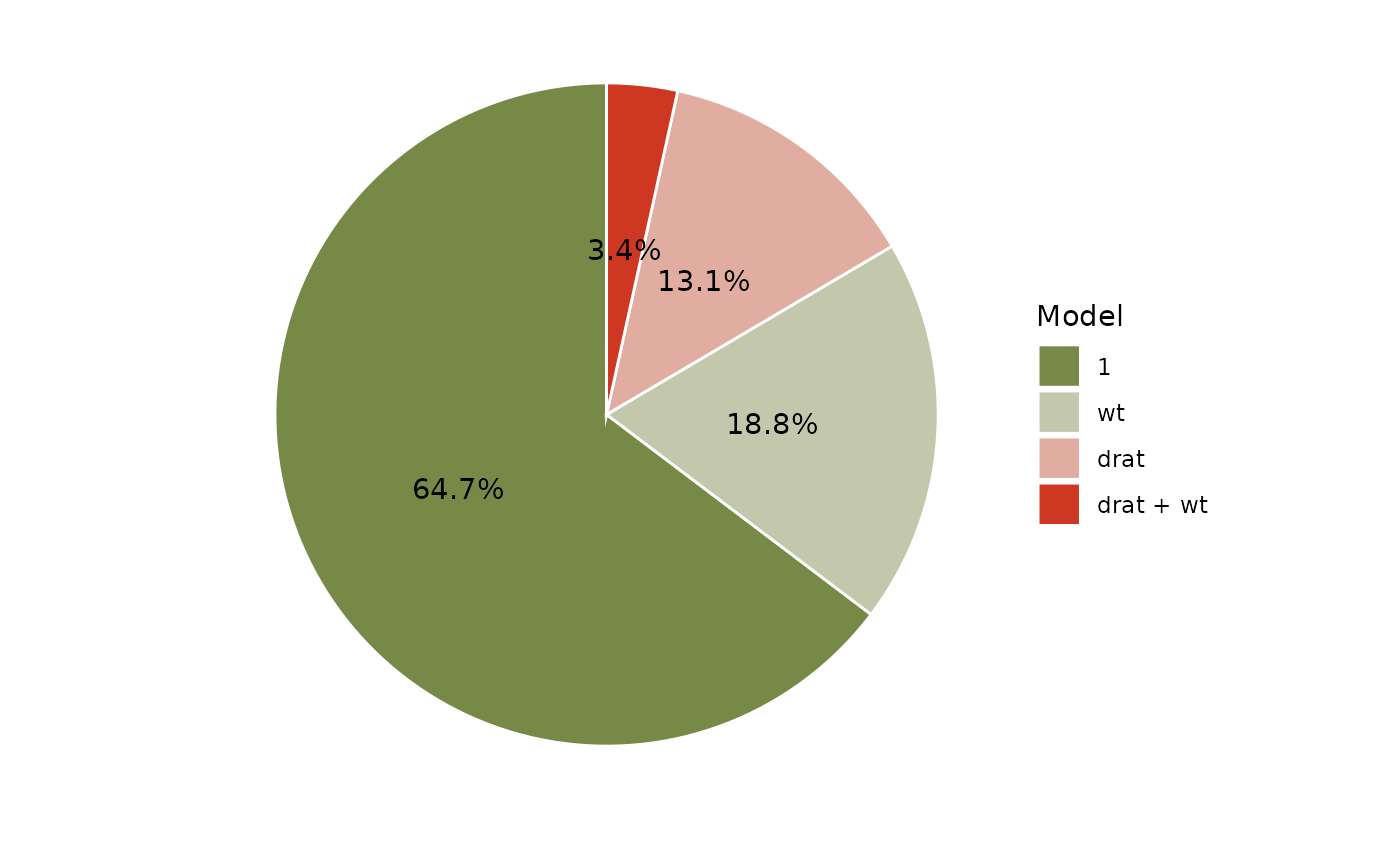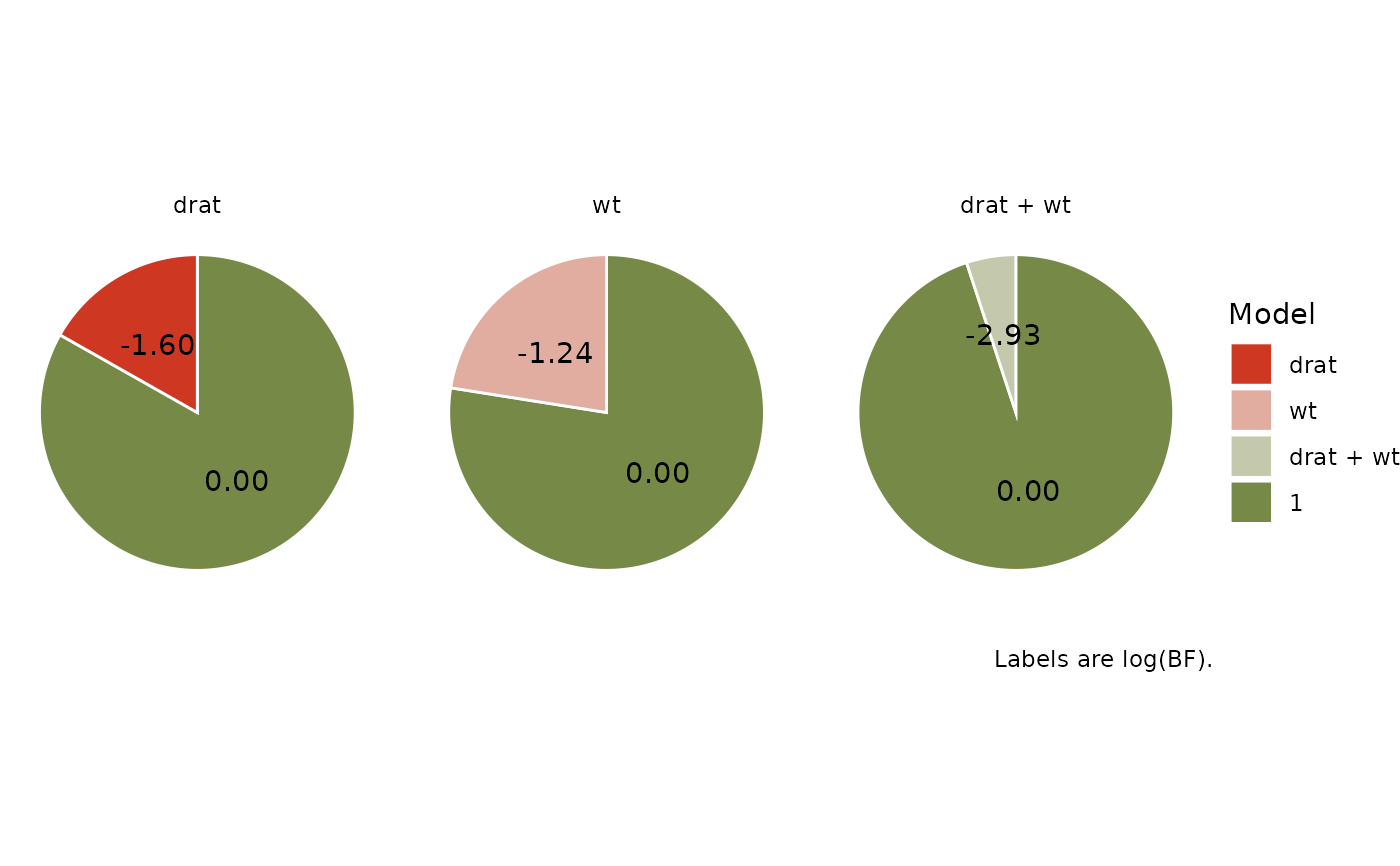The plot() method for the bayestestR::bayesfactor_models() function. These plots visualize the posterior probabilities of the compared models.

## Usage

# S3 method for see_bayesfactor_models
plot(
x,
n_pies = c("one", "many"),
value = c("none", "BF", "probability"),
sort = FALSE,
log = FALSE,
prior_odds = NULL,
...
)

## Arguments

x

An object.

n_pies

Number of pies.

value

What value to display.

sort

The behavior of this argument depends on the plotting contexts.

• Plotting model parameters: If NULL, coefficients are plotted in the order as they appear in the summary. Setting sort = "ascending" or sort = "descending" sorts coefficients in ascending or descending order, respectively. Setting sort = TRUE is the same as sort = "ascending".

• Plotting Bayes factors: Sort pie-slices by posterior probability (descending)?

log

Logical that decides whether to display log-transformed Bayes factors.

prior_odds

An optional vector of prior odds for the models. See BayesFactor::priorOdds. As the size of the pizza slices corresponds to posterior probability (which is a function of prior probability and the Bayes Factor), custom prior_odds will change the slices' size.

...

Arguments passed to or from other methods.

## Value

A ggplot2-object.

## Examples

library(bayestestR)
library(see)

lm0 <- lm(qsec ~ 1, data = mtcars)
lm1 <- lm(qsec ~ drat, data = mtcars)
lm2 <- lm(qsec ~ wt, data = mtcars)
lm3 <- lm(qsec ~ drat + wt, data = mtcars)

result <- bayesfactor_models(lm1, lm2, lm3, denominator = lm0)

plot(result, n_pies = "one", value = "probability", sort = TRUE) +
scale_fill_pizza(reverse = TRUE)plot(result, n_pies = "many", value = "BF", log = TRUE) +
scale_fill_pizza(reverse = FALSE)